# Stuck on this problem, an explanation of the answer would be very much appreciated!!

Stuck on this problem, an explanation of the answer would be very much appreciated!!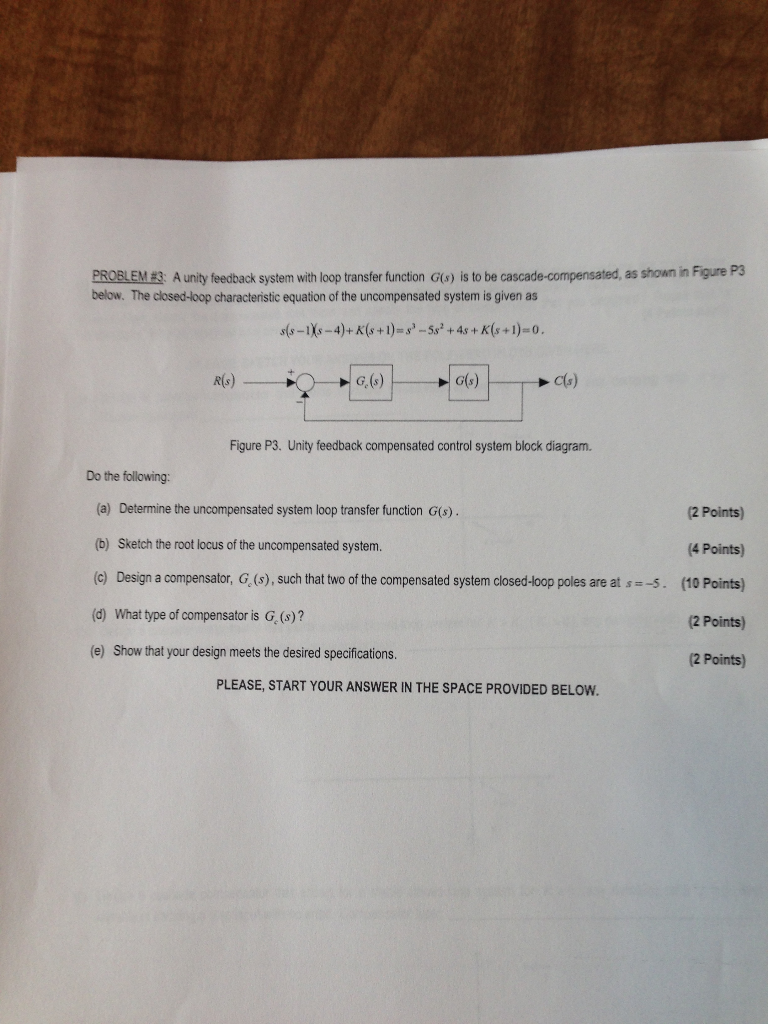PROBLEM E3 A unity feedback system with loop transfer function G(o) is to be cascade-compensated, as shown in Figure P3 below. The closed-loop characteristic equation of the uncompensated system is given as G. (s) G(s) Cs) Figure P3. Unity feedback compensated control system block diagram. Do the following: (a) Determine the uncompensated system loop transfer function G(s). b) Sketch the root locus of the uncompensated system. (c) Design a compensator, G, (s), such that two of the compensated system closed-loop poles are at s 5. (10 Points) d) What type of compensator is G. (s)? (e) Show that your design meets the desired specifications. 2 Points) (4 Points) 2 Points) 2 Points) PLEASE, START YOUR ANSWER IN THE SPACE PROVIDED BELOW##### Add Answer of: Stuck on this problem, an explanation of the answer would be very much appreciated!!
Similar Homework Help Questions
• ### Problem 4. The open-loop transfer function of a unity feedback system is 20 G(s) S+1.5) (s +3.5) ...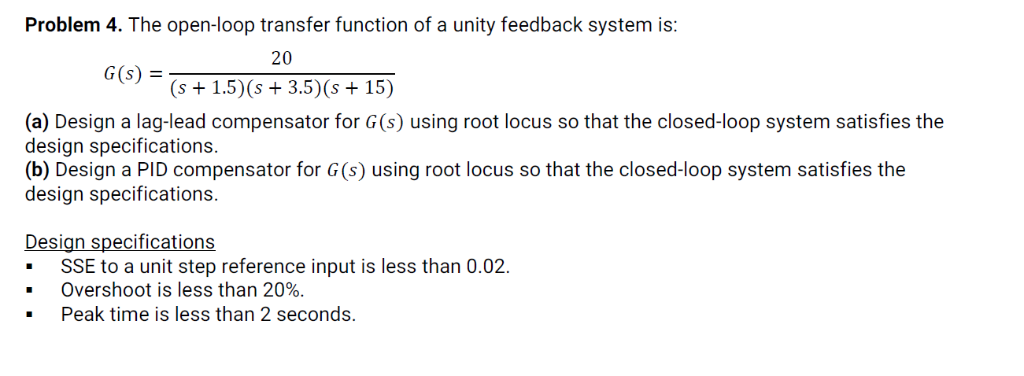Problem 4. The open-loop transfer function of a unity feedback system is 20 G(s) S+1.5) (s +3.5) (s +15) (a) Design a lag-lead compensator for G(s) using root locus so that the closed-loop system satisfies the design specifications. (b) Design a PID compensator for G(s) using root locus so that the closed-loop system satisfies the design specifications. Design specifications -SSE to a unit step reference input is less than 0.02. Overshoot is less than 20%. Peak time is less than...

• ### Problem 4. The open-loop transfer function of a unity feedback system is: 20 (s+1.5)(s 3.5) (s 15...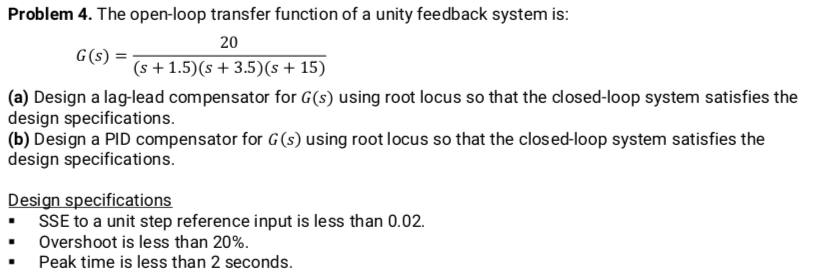Problem 4. The open-loop transfer function of a unity feedback system is: 20 (s+1.5)(s 3.5) (s 15) G(s) (a) Design a lag-lead compensator for G(s) using root locus so that the closed-loop system satisfies the design specifications (b) Design a PID compensator for G (s) using root locus so that the clos ed-loop system satisfies the design specifications. Design specifications .SSE to a unit step reference input is less than 0.02. Overshoot is less than 20% Peak time is less...

• ### 3 .0.2) 0(z) ℉.20-1011,. +20s+101)(s+20) 20), the damping ratio for the dominant Problem 2: For a...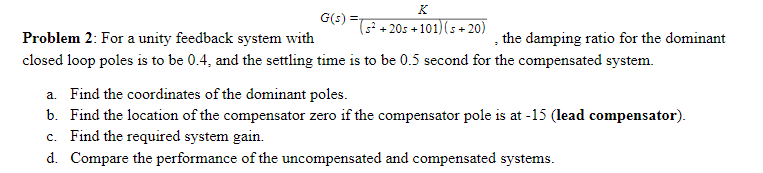3 .0.2) 0(z) ℉.20-1011,. +20s+101)(s+20) 20), the damping ratio for the dominant Problem 2: For a unity feedback system with closed loop poles is to be 0.4, and the settling time is to be 0.5 second for the compensated system. a. b. c. d. Find the coordinates of the dominant poles. Find the location of the compensator zero if the compensator pole is at -15 (lead compensator) Find the required system gain. Compare the performance of the uncompensated and compensated...

• ### A plant with the transfer function Gp(s)-- with unity feedback has the root locus shown in the figure below: (s+2)(s+4) Root Locus 1.5 C(s) 0.5 0.5 1.5 .3 Real Axis (seconds) (a) Determine K of G...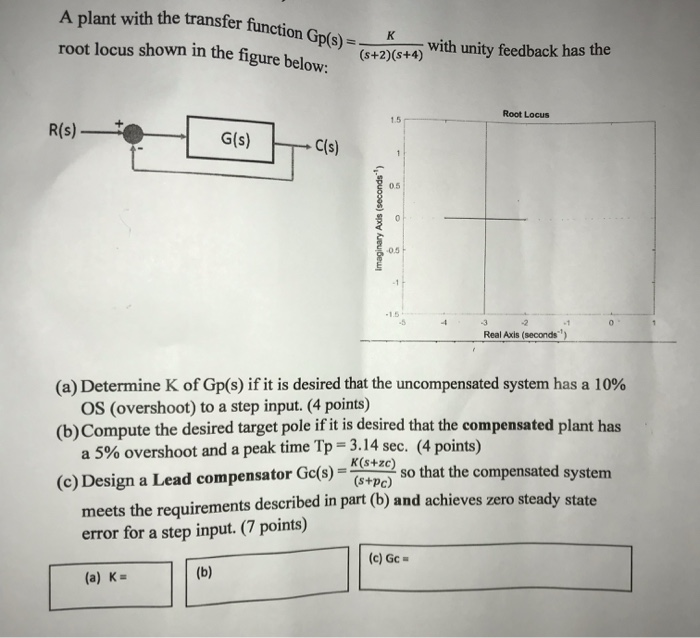A plant with the transfer function Gp(s)-- with unity feedback has the root locus shown in the figure below: (s+2)(s+4) Root Locus 1.5 C(s) 0.5 0.5 1.5 .3 Real Axis (seconds) (a) Determine K of Gp(s) if it is desired that the uncompensated system has a 10% OS (overshoot) to a step input. (4 points) a 5% overshoot and a peak time Tp 3.1 meets the requirements described in part (b) and achieves zero steady state (b) Compute the desired...

• ### 4) 3s points 11. Given the unity feedback system of Figure P9.1 with G(s) K (s + 6) do the following: [Section: 9.3 a. Sketch the root locus. b) Using the operating point of -3.2+j2.38 find the g...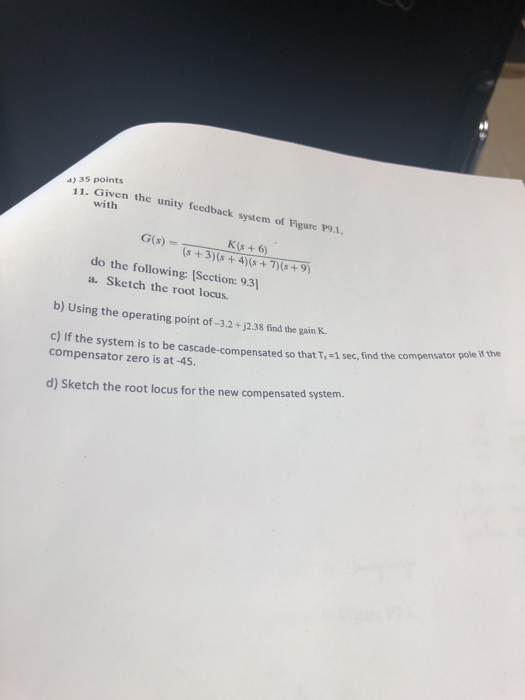4) 3s points 11. Given the unity feedback system of Figure P9.1 with G(s) K (s + 6) do the following: [Section: 9.3 a. Sketch the root locus. b) Using the operating point of -3.2+j2.38 find the gain K. c) if the system is to be cascade-compensated so that T, -1 sec, find the compensator compensator zero is at -45. pole if the d) Sketch the root locus for the new compensated system. 4) 3s points 11. Given the unity...

• ### 1. Given a unity feedback system with the open-loop transfer function s(0.5s +1) .design a lead c...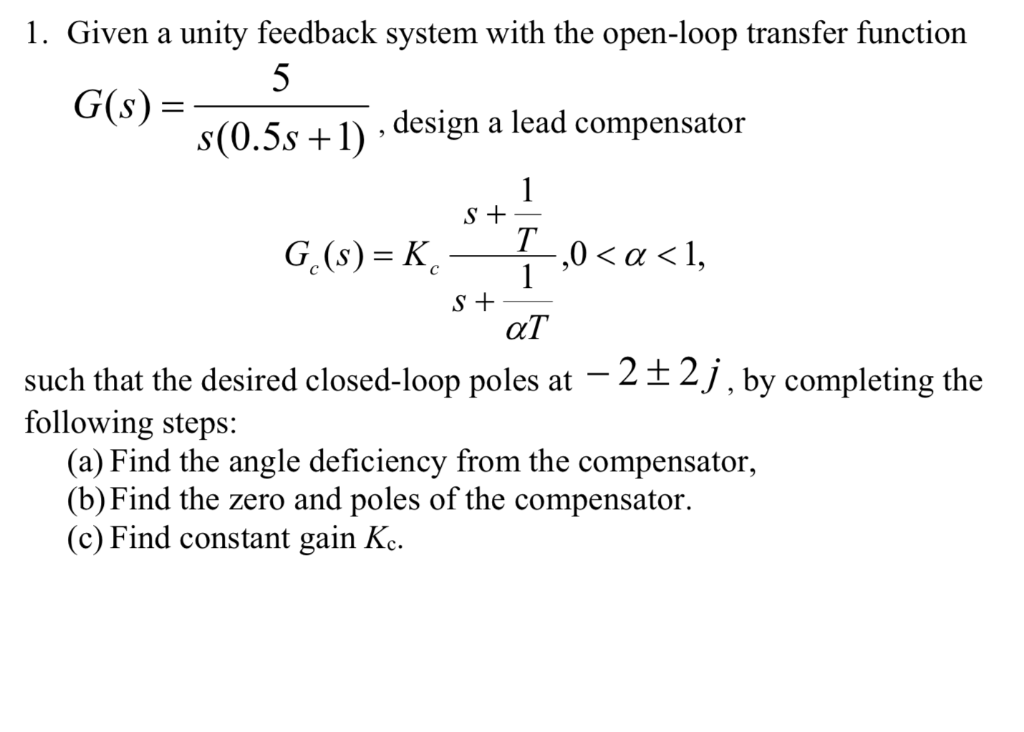1. Given a unity feedback system with the open-loop transfer function s(0.5s +1) .design a lead compensator ,0 〈 α 〈 1, such that the desired closed-loop poles at -2+2j following steps: J, by completing the (a) Find the angle deficiency from the compensator, (b)Find the zero and poles of the compensator (c) Find constant gain Kc. 1. Given a unity feedback system with the open-loop transfer function s(0.5s +1) .design a lead compensator ,0 〈 α 〈 1, such...

• ### Please solve parts (a) and (b) neatly and show problem solving. Ignore reference to part 1, but please still plot the root loci. For the system given in Figure 1 a) Design a PD compensator with the t...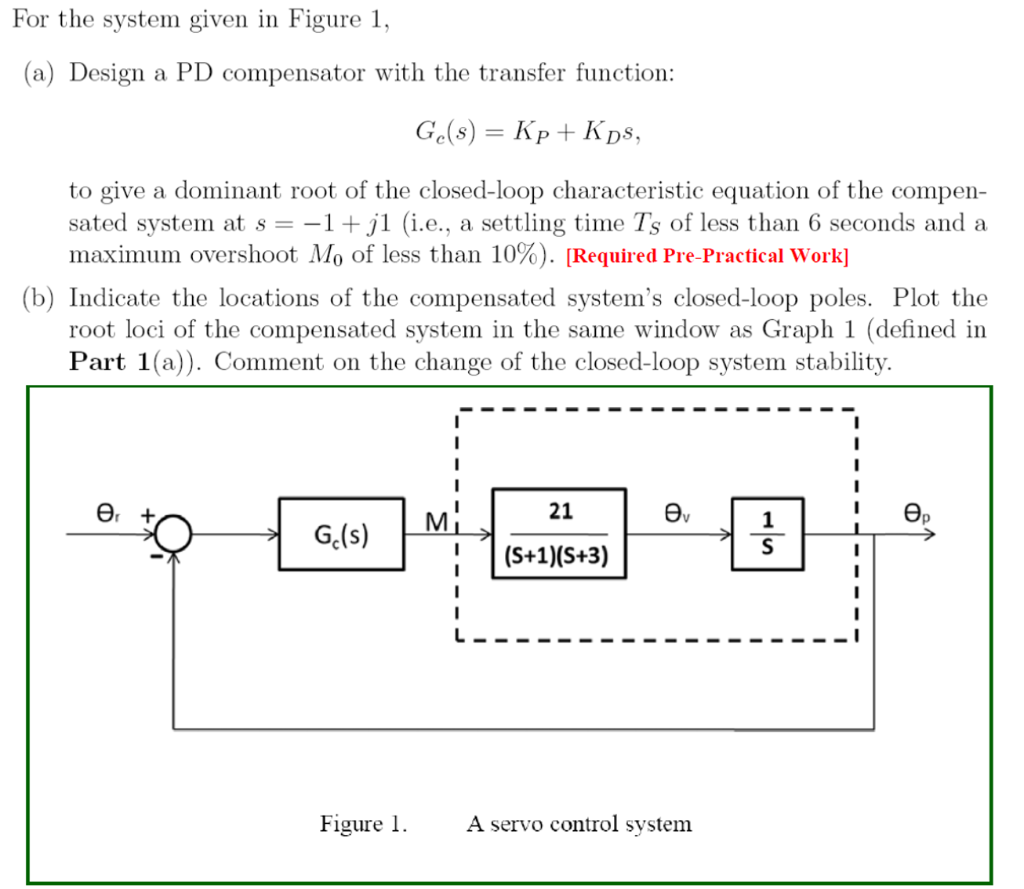Please solve parts (a) and (b) neatly and show problem solving. Ignore reference to part 1, but please still plot the root loci. For the system given in Figure 1 a) Design a PD compensator with the transfer function: to give a dominant root of the closed-loop characteristic equation of the compen- sated system at s -1+j1 (i.e., a settling time Ts of less than 6 seconds and a maximum overshoot Mo of less than 10%). Required Pre-Practical work] (b)...

• ### Problem 2 For the unity feedback system below in Figure 2 G(s) Figure 2. With (8+2) G(s) = (a) Sk...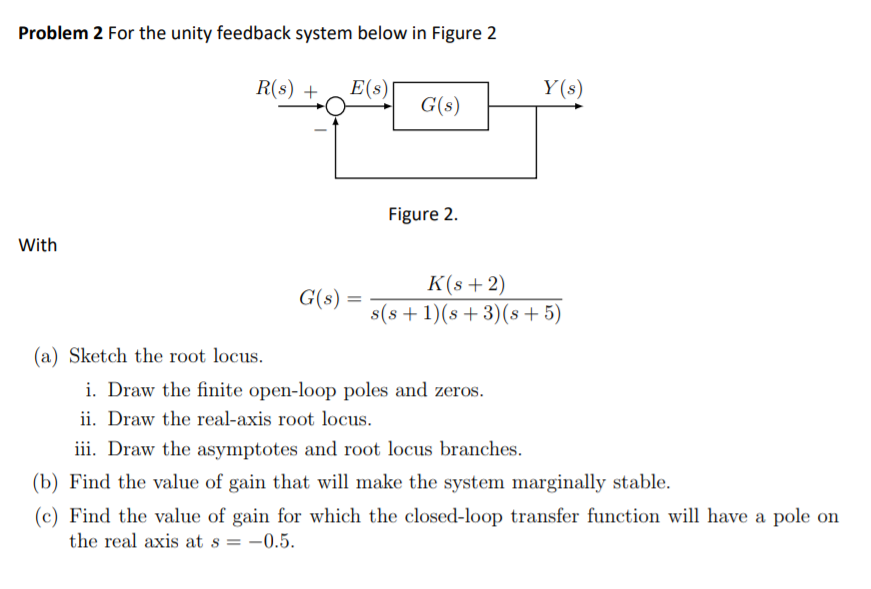Problem 2 For the unity feedback system below in Figure 2 G(s) Figure 2. With (8+2) G(s) = (a) Sketch the root locus. 1. Draw the finite open-loop poles and zeros. ii. Draw the real-axis root locus iii. Draw the asymptotes and root locus branches. (b) Find the value of gain that will make the system marginally stable. (c) Find the value of gain for which the closed-loop transfer function will have a pole on the real axis at s...

• ### Question 1 (60 points) Consider the following block diagram where G(s)- Controller R(s) G(s) (a) Sketch the root locus assuming a proportional controller is used. [25 points] (b) Design specifica...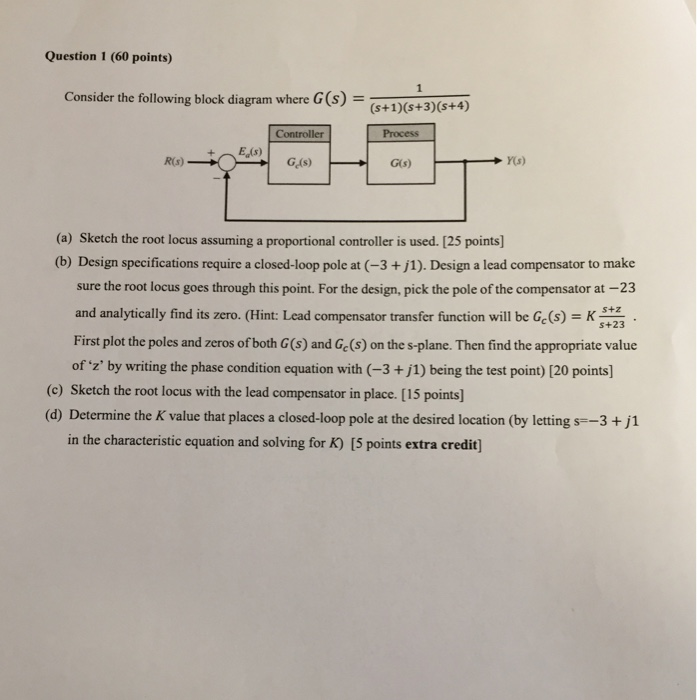Question 1 (60 points) Consider the following block diagram where G(s)- Controller R(s) G(s) (a) Sketch the root locus assuming a proportional controller is used. [25 points] (b) Design specifications require a closed-loop pole at (-3+j1). Design a lead compensator to make sure the root locus goes through this point. For the design, pick the pole of the compensator at-23 and analytically find its zero. (Hint: Lead compensator transfer function will be Ge (s)\$+23 First plot the poles and zeros...

• ### USING MATLAB TO SOLVE THIS PROBLEM - Figure Above ---------------------------------------------------------------------...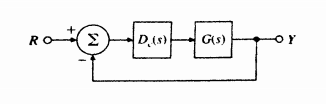USING MATLAB TO SOLVE THIS PROBLEM - Figure Above ---------------------------------------------------------------------- We were unable to transcribe this image26. A numerically controlled machine tool positioning servomechanism has a normalized and scaled transfer function given by S(s+1 Performance specifications of the system in the unity feedback configu ration of Fig. 5.60 are satisfied if the closed-loop poles are located at (a) Show that this specification cannot be achieved by choosing propor tional control alone, D(s) = a lead compensator D)- that will s...

Need Online Homework Help?